In :
%load_ext autoreload
%matplotlib inline
import random
random.seed(1100038344)
import survivalstan
import numpy as np
import pandas as pd
from stancache import stancache
from matplotlib import pyplot as plt

INFO:stancache.seed:Setting seed to 1245502385

In :
model_code = survivalstan.models.pem_survival_model_randomwalk

In :
print(model_code)

/*  Variable naming:
// dimensions
N          = total number of observations (length of data)
S          = number of sample ids
T          = max timepoint (number of timepoint ids)
M          = number of covariates

// main data matrix (per observed timepoint*record)
s          = sample id for each obs
t          = timepoint id for each obs
event      = integer indicating if there was an event at time t for sample s
x          = matrix of real-valued covariates at time t for sample n [N, X]

// timepoint-specific data (per timepoint, ordered by timepoint id)
t_obs      = observed time since origin for each timepoint id (end of period)
t_dur      = duration of each timepoint period (first diff of t_obs)

*/
// Jacqueline Buros Novik <[email protected]>

data {
// dimensions
int<lower=1> N;
int<lower=1> S;
int<lower=1> T;
int<lower=0> M;

// data matrix
int<lower=1, upper=N> s[N];     // sample id
int<lower=1, upper=T> t[N];     // timepoint id
int<lower=0, upper=1> event[N]; // 1: event, 0:censor
matrix[N, M] x;                 // explanatory vars

// timepoint data
vector<lower=0>[T] t_obs;
vector<lower=0>[T] t_dur;
}
transformed data {
vector[T] log_t_dur;  // log-duration for each timepoint
int n_trans[S, T];

log_t_dur = log(t_obs);

// n_trans used to map each sample*timepoint to n (used in gen quantities)
// map each patient/timepoint combination to n values
for (n in 1:N) {
n_trans[s[n], t[n]] = n;
}

// fill in missing values with n for max t for that patient
// ie assume "last observed" state applies forward (may be problematic for TVC)
// this allows us to predict failure times >= observed survival times
for (samp in 1:S) {
int last_value;
last_value = 0;
for (tp in 1:T) {
// manual says ints are initialized to neg values
// so <=0 is a shorthand for "unassigned"
if (n_trans[samp, tp] <= 0 && last_value != 0) {
n_trans[samp, tp] = last_value;
} else {
last_value = n_trans[samp, tp];
}
}
}
}
parameters {
vector[T] log_baseline_raw; // unstructured baseline hazard for each timepoint t
vector[M] beta;                      // beta for each covariate
real<lower=0> baseline_sigma;
real log_baseline_mu;
}
transformed parameters {
vector[N] log_hazard;
vector[T] log_baseline;

log_baseline = log_baseline_raw + log_t_dur;

for (n in 1:N) {
log_hazard[n] = log_baseline_mu + log_baseline[t[n]] + x[n,]*beta;
}
}
model {
beta ~ cauchy(0, 2);
event ~ poisson_log(log_hazard);
log_baseline_mu ~ normal(0, 1);
baseline_sigma ~ normal(0, 1);
log_baseline_raw ~ normal(0, 1);
for (i in 2:T) {
log_baseline_raw[i] ~ normal(log_baseline_raw[i-1], baseline_sigma);
}
}
generated quantities {
real log_lik[N];
vector[T] baseline;
int y_hat_mat[S, T];     // ppcheck for each S*T combination
real y_hat_time[S];      // predicted failure time for each sample
int y_hat_event[S];      // predicted event (0:censor, 1:event)

// compute raw baseline hazard, for summary/plotting
baseline = exp(log_baseline_raw);

for (n in 1:N) {
log_lik[n] <- poisson_log_lpmf(event[n] | log_hazard[n]);
}

// posterior predicted values
for (samp in 1:S) {
int sample_alive;
sample_alive = 1;
for (tp in 1:T) {
if (sample_alive == 1) {
int n;
int pred_y;
real log_haz;

// determine predicted value of y
// (need to recalc so that carried-forward data use sim tp and not t[n])
n = n_trans[samp, tp];
log_haz = log_baseline_mu + log_baseline[tp] + x[n,]*beta;
if (log_haz < log(pow(2, 30)))
pred_y = poisson_log_rng(log_haz);
else
pred_y = 9;

// mark this patient as ineligible for future tps
// note: deliberately make 9s ineligible
if (pred_y >= 1) {
sample_alive = 0;
y_hat_time[samp] = t_obs[tp];
y_hat_event[samp] = 1;
}

// save predicted value of y to matrix
y_hat_mat[samp, tp] = pred_y;
}
else if (sample_alive == 0) {
y_hat_mat[samp, tp] = 9;
}
} // end per-timepoint loop

// if patient still alive at max
//
if (sample_alive == 1) {
y_hat_time[samp] = t_obs[T];
y_hat_event[samp] = 0;
}
} // end per-sample loop
}


In :
d = stancache.cached(
survivalstan.sim.sim_data_exp_correlated,
N=100,
censor_time=20,
rate_form='1 + sex',
rate_coefs=[-3, 0.5],
)
d['age_centered'] = d['age'] - d['age'].mean()

INFO:stancache.stancache:sim_data_exp_correlated: cache_filename set to sim_data_exp_correlated.cached.N_100.censor_time_20.rate_coefs_54462717316.rate_form_1 + sex.pkl

Out:
sex age rate true_t t event index age_centered
0 male 54 0.082085 1.013855 1.013855 True 0 -1.12
1 male 39 0.082085 4.890597 4.890597 True 1 -16.12
2 female 45 0.049787 4.093404 4.093404 True 2 -10.12
3 female 43 0.049787 7.036226 7.036226 True 3 -12.12
4 female 57 0.049787 5.712299 5.712299 True 4 1.88
In :
survivalstan.utils.plot_observed_survival(df=d[d['sex']=='female'], event_col='event', time_col='t', label='female')
survivalstan.utils.plot_observed_survival(df=d[d['sex']=='male'], event_col='event', time_col='t', label='male')
plt.legend()

Out:
<matplotlib.legend.Legend at 0x7f91143b96a0>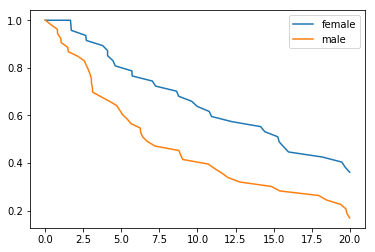In :
dlong = stancache.cached(
survivalstan.prep_data_long_surv,
df=d, event_col='event', time_col='t'
)

INFO:stancache.stancache:prep_data_long_surv: cache_filename set to prep_data_long_surv.cached.df_14209590808.event_col_event.time_col_t.pkl

In :
dlong.head()

Out:
sex age rate true_t t event index age_centered end_time end_failure
0 male 54 0.082085 1.013855 1.013855 True 0 -1.12 1.013855 True
58 male 54 0.082085 1.013855 1.013855 True 0 -1.12 0.808987 False
65 male 54 0.082085 1.013855 1.013855 True 0 -1.12 0.377535 False
72 male 54 0.082085 1.013855 1.013855 True 0 -1.12 0.791192 False
73 male 54 0.082085 1.013855 1.013855 True 0 -1.12 0.009787 False
In :
testfit = survivalstan.fit_stan_survival_model(
model_cohort = 'test model',
model_code = model_code,
df = dlong,
sample_col = 'index',
timepoint_end_col = 'end_time',
event_col = 'end_failure',
formula = '~ age_centered + sex',
iter = 5000,
chains = 4,
seed = 9001,
FIT_FUN = stancache.cached_stan_fit,
)

INFO:stancache.stancache:Step 1: Get compiled model code, possibly from cache
INFO:stancache.stancache:StanModel: cache_filename set to anon_model.cython_0_29_2.model_code_17281568671805165521.pystan_2_18_1_0.stanmodel.pkl
INFO:stancache.stancache:Step 2: Get posterior draws from model, possibly from cache
INFO:stancache.stancache:sampling: cache_filename set to anon_model.cython_0_29_2.model_code_17281568671805165521.pystan_2_18_1_0.stanfit.chains_4.data_75284643319.iter_5000.seed_9001.pkl

In :
survivalstan.utils.print_stan_summary([testfit], pars='lp__')

            mean   se_mean         sd        2.5%        50%       97.5%      Rhat
lp__ -287.781216  1.521948  25.841239 -337.843663 -288.32069 -236.827395  1.006161

In :
survivalstan.utils.print_stan_summary([testfit], pars='log_baseline_raw')

                          mean   se_mean        sd      2.5%       50%     97.5%      Rhat
log_baseline_raw  -2.266052  0.022797  0.757871 -3.781383 -2.264353 -0.801449  1.002332
log_baseline_raw  -2.364431  0.022200  0.747522 -3.852205 -2.352824 -0.913209  1.002224
log_baseline_raw  -2.469519  0.022284  0.742275 -3.943979 -2.457068 -1.035849  1.001857
log_baseline_raw  -2.566836  0.022849  0.744848 -4.040161 -2.548941 -1.108471  1.001946
log_baseline_raw  -2.662735  0.023252  0.749227 -4.152525 -2.639411 -1.206405  1.001873
log_baseline_raw  -2.754253  0.024026  0.759507 -4.251543 -2.735682 -1.266185  1.001951
log_baseline_raw  -2.843475  0.024597  0.768474 -4.378099 -2.824548 -1.343800  1.001972
log_baseline_raw  -2.924604  0.025082  0.776434 -4.479474 -2.907346 -1.419350  1.002177
log_baseline_raw  -3.003173  0.025526  0.782436 -4.581052 -2.986473 -1.474753  1.002179
log_baseline_raw -3.073193  0.026218  0.791575 -4.648971 -3.066341 -1.511074  1.002226
log_baseline_raw -3.139566  0.026516  0.796587 -4.732211 -3.129224 -1.578292  1.002020
log_baseline_raw -3.209124  0.026946  0.799748 -4.801769 -3.197802 -1.646758  1.001927
log_baseline_raw -3.270523  0.027736  0.804584 -4.867368 -3.257556 -1.714762  1.001913
log_baseline_raw -3.324910  0.028008  0.806302 -4.952066 -3.322625 -1.770464  1.002156
log_baseline_raw -3.375416  0.028060  0.808821 -4.986150 -3.358505 -1.787048  1.002112
log_baseline_raw -3.418015  0.028232  0.809641 -5.020435 -3.403251 -1.825160  1.002190
log_baseline_raw -3.460291  0.028885  0.812362 -5.056463 -3.444036 -1.880696  1.002066
log_baseline_raw -3.498345  0.028933  0.812367 -5.117012 -3.495259 -1.892893  1.002061
log_baseline_raw -3.535051  0.028993  0.814328 -5.132985 -3.522936 -1.952877  1.002407
log_baseline_raw -3.568242  0.028936  0.815294 -5.190021 -3.565835 -1.994228  1.002462
log_baseline_raw -3.596620  0.028745  0.812500 -5.214018 -3.583318 -2.004260  1.002160
log_baseline_raw -3.627828  0.028897  0.816340 -5.249507 -3.621776 -2.027126  1.002112
log_baseline_raw -3.664812  0.028823  0.817502 -5.285838 -3.660719 -2.057037  1.002035
log_baseline_raw -3.694122  0.028644  0.816023 -5.313456 -3.679695 -2.101070  1.001962
log_baseline_raw -3.726449  0.028639  0.817342 -5.351640 -3.718952 -2.122747  1.001737
log_baseline_raw -3.754691  0.028893  0.819345 -5.393803 -3.751139 -2.151594  1.001739
log_baseline_raw -3.783266  0.028977  0.821166 -5.411232 -3.778064 -2.168727  1.001889
log_baseline_raw -3.808153  0.028980  0.821477 -5.440836 -3.796426 -2.203063  1.001925
log_baseline_raw -3.835505  0.028949  0.821662 -5.474397 -3.827508 -2.242330  1.002038
log_baseline_raw -3.860725  0.028877  0.817381 -5.478707 -3.848681 -2.272787  1.002150
log_baseline_raw -3.885441  0.028842  0.816953 -5.513805 -3.874989 -2.308918  1.002068
log_baseline_raw -3.908965  0.029221  0.816717 -5.532251 -3.896064 -2.326129  1.001938
log_baseline_raw -3.931273  0.029425  0.818813 -5.564209 -3.914277 -2.364230  1.001907
log_baseline_raw -3.953029  0.029330  0.820281 -5.578620 -3.943326 -2.385194  1.001686
log_baseline_raw -3.973201  0.029288  0.821152 -5.607299 -3.967871 -2.381836  1.001760
log_baseline_raw -3.995195  0.029151  0.819997 -5.641221 -3.982282 -2.410138  1.001859
log_baseline_raw -4.013542  0.028848  0.821193 -5.670351 -4.006696 -2.440001  1.001911
log_baseline_raw -4.032756  0.028732  0.821102 -5.672715 -4.031058 -2.435735  1.001869
log_baseline_raw -4.054814  0.028657  0.821730 -5.677297 -4.052578 -2.462860  1.002034
log_baseline_raw -4.077615  0.029048  0.820922 -5.714226 -4.078718 -2.484970  1.002175
log_baseline_raw -4.100401  0.029174  0.822995 -5.752051 -4.096290 -2.507281  1.002144
log_baseline_raw -4.120889  0.029157  0.823284 -5.771448 -4.114912 -2.524585  1.002094
log_baseline_raw -4.139251  0.029130  0.822729 -5.767588 -4.132056 -2.544751  1.001926
log_baseline_raw -4.163014  0.029071  0.821213 -5.812907 -4.159177 -2.570496  1.002177
log_baseline_raw -4.179724  0.028949  0.820050 -5.835372 -4.163769 -2.615221  1.002185
log_baseline_raw -4.197610  0.028892  0.822176 -5.823872 -4.189961 -2.597658  1.002236
log_baseline_raw -4.212008  0.029008  0.819808 -5.849129 -4.202241 -2.633780  1.002305
log_baseline_raw -4.225084  0.028957  0.818729 -5.848362 -4.215952 -2.643008  1.001915
log_baseline_raw -4.241355  0.029079  0.818991 -5.863295 -4.227776 -2.670875  1.001969
log_baseline_raw -4.256881  0.029008  0.818179 -5.891835 -4.254293 -2.664914  1.002075
log_baseline_raw -4.270769  0.029369  0.818646 -5.904414 -4.265443 -2.694613  1.002035
log_baseline_raw -4.282680  0.029372  0.818056 -5.931432 -4.278622 -2.698225  1.001975
log_baseline_raw -4.294047  0.029321  0.816835 -5.942168 -4.289579 -2.713233  1.002032
log_baseline_raw -4.305103  0.029514  0.818933 -5.933277 -4.298894 -2.706766  1.001916
log_baseline_raw -4.312760  0.029303  0.818738 -5.933212 -4.312261 -2.728168  1.001963
log_baseline_raw -4.324978  0.029258  0.820226 -5.964142 -4.319273 -2.726846  1.001836
log_baseline_raw -4.337596  0.029456  0.822980 -5.957788 -4.334267 -2.729425  1.001769
log_baseline_raw -4.350086  0.029573  0.823873 -5.990426 -4.349105 -2.740828  1.001933
log_baseline_raw -4.363347  0.029452  0.820318 -5.998213 -4.363577 -2.763006  1.001743
log_baseline_raw -4.376516  0.029280  0.821438 -6.019750 -4.373609 -2.763041  1.001752
log_baseline_raw -4.381173  0.029498  0.821282 -6.026957 -4.378750 -2.777419  1.001712
log_baseline_raw -4.390519  0.029471  0.821330 -6.018604 -4.387542 -2.796227  1.001823
log_baseline_raw -4.394140  0.029188  0.822449 -6.040613 -4.391103 -2.784246  1.001807
log_baseline_raw -4.400284  0.029012  0.820515 -6.045551 -4.401524 -2.807080  1.001888
log_baseline_raw -4.410931  0.029522  0.823018 -6.024781 -4.408396 -2.811094  1.001875
log_baseline_raw -4.417436  0.029669  0.823858 -6.064181 -4.411878 -2.834595  1.001887
log_baseline_raw -4.427447  0.029746  0.825849 -6.073543 -4.427379 -2.846127  1.001931
log_baseline_raw -4.438940  0.030008  0.828293 -6.087548 -4.432626 -2.846181  1.001923
log_baseline_raw -4.450288  0.030352  0.833324 -6.133451 -4.438808 -2.833132  1.002091
log_baseline_raw -4.459671  0.030378  0.836460 -6.138809 -4.454855 -2.830563  1.002030
log_baseline_raw -4.470068  0.030428  0.839366 -6.146245 -4.471940 -2.844583  1.001988
log_baseline_raw -4.481865  0.030131  0.841145 -6.155597 -4.480798 -2.860652  1.001826
log_baseline_raw -4.496755  0.030241  0.853279 -6.209481 -4.500568 -2.843853  1.001606
log_baseline_raw -4.515678  0.030588  0.864968 -6.246099 -4.505534 -2.848791  1.001712
log_baseline_raw -4.541684  0.031342  0.883239 -6.323431 -4.536108 -2.856660  1.001737

In :
survivalstan.utils.plot_stan_summary([testfit], pars='log_baseline_raw')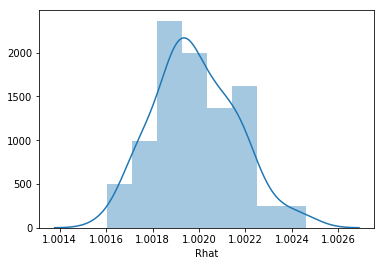In :
survivalstan.utils.plot_coefs([testfit], element='baseline')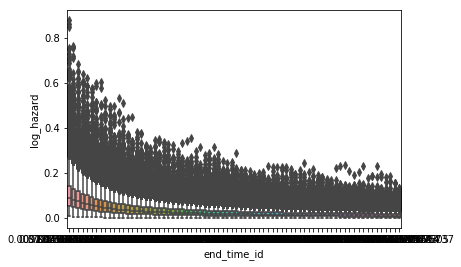In :
survivalstan.utils.plot_coefs([testfit])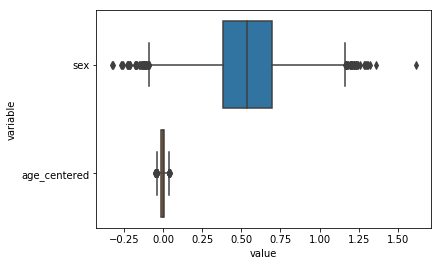In :
survivalstan.utils.plot_pp_survival([testfit], fill=False)
survivalstan.utils.plot_observed_survival(df=d, event_col='event', time_col='t', color='green', label='observed')
plt.legend()

Out:
<matplotlib.legend.Legend at 0x7f9024bef470>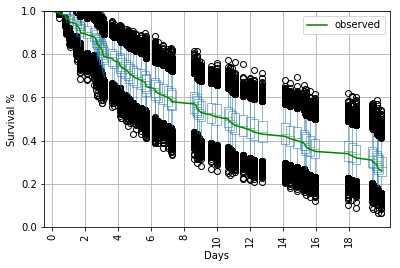In :
survivalstan.utils.plot_pp_survival([testfit], by='sex')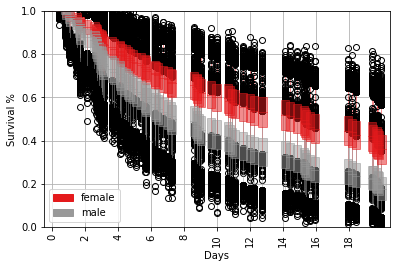In [ ]:


In [ ]: